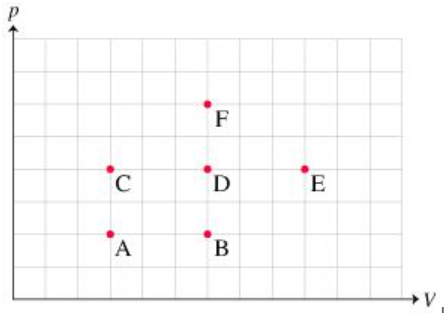# Problem: Ideal Gas Law and Internal Energy Pressure vs. Volume Graph Ranking Task:(a) Rank the states on the basis of the average kinetic energy of the atoms in the gas sample at each state.(b) Rank from largest to smallest. To rank items as equivalent, overlap them.

###### FREE Expert Solution

The ideal gas equation is:

$\overline{){\mathbf{P}}{\mathbf{V}}{\mathbf{=}}{\mathbf{n}}{\mathbf{RT}}}$

Kinetic energy is directly proportional to temperature.

The greatest product PV will have the greatest temperature, and hence the largest kinetic energy.

A. PV = (2)(3) = 6

B. PV = (2)(6) = 12

C. PV = (4)(3) = 12

95% (468 ratings)###### Problem Details

Ideal Gas Law and Internal Energy Pressure vs. Volume Graph Ranking Task:

(a) Rank the states on the basis of the average kinetic energy of the atoms in the gas sample at each state.

(b) Rank from largest to smallest. To rank items as equivalent, overlap them.